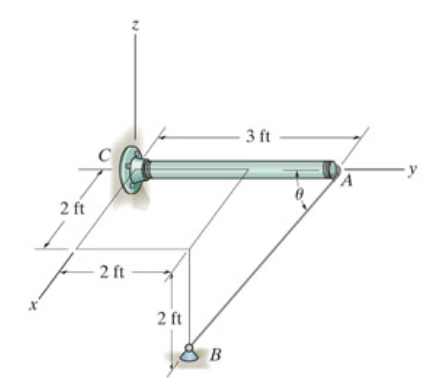# Problem: Determine the angle θ between the y axis of the pole and the wire AB.Express your answer to three significant figures and include the appropriate units.

###### FREE Expert Solution

In this problem, we first need to write the coordinates of the points A, B, and C.

A = (0, 3, 0)

B = (2, 2, -2)

C = (0, 0, 0)

100% (19 ratings)###### Problem DetailsDetermine the angle θ between the y axis of the pole and the wire AB.
Express your answer to three significant figures and include the appropriate units.